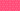PricingResources

# What is intrinsic value?

Written by

There are numerous factors involved when assessing the value of any asset. One is its intrinsic value, which can be calculated either through objective analysis or a financial model. Here’s how it works.

## Intrinsic value definition

Intrinsic value is a term that describes what an asset is worth, but this can have slightly different applications. In options pricing, intrinsic value marks the difference between the asset’s current price and option strike price. In financial analysis, it’s used to work out a company’s underlying value according to the current market.

In both cases, it uses a financial model or objective calculation to determine a figure of worth, rather than relying on the asset’s current trading price.

## Why is intrinsic value useful?

Investors can make decisions based on current stock prices alone, but this doesn’t paint the full picture of an asset’s worth. With intrinsic value, investors are able to determine what the stock is really worth. This is particularly helpful for value investors who seek out undervalued stocks or other discounted investment options.

## How to calculate intrinsic value

There are several calculation methods to choose from when working out an asset’s intrinsic value.

### Discounted cash flow analysis

A discounted cash flow analysis (DCF) dives into a company’s cash flows to arrive at intrinsic value. There are three steps involved:

1. Estimate future cash flows.

2. Calculate the present value of future cash flows.

3. Analyse present value to obtain intrinsic value.

There’s no way to estimate a company’s future cash flows with 100% accuracy, but its financial statements are a reliable place to start. Investors will also need to research the company’s growth prospects.

The DCF method looks at the company’s weighted average cost of capital (WACC) to discount future cash flows. WACC is the expected rate of return, which accounts for the time value of money. Using WACC, you can complete step two to bring future cash flows into the present. With those figures in hand, you can use the DCF model to estimate future revenue streams and rates of return. These give you an insight into whether the investment is worth pursuing.

### Financial Metric Analysis

A second way to work out intrinsic value is by applying a financial metric, like the price-to-earnings ratio. In this case, where r = expected earnings growth rate:

### Asset-based valuation

A third option is to use an asset-based valuation to calculate a stock’s intrinsic value. The intrinsic value formula for this method is perhaps the simplest of the three:

Company assets would include both tangible and intangible assets, while liabilities would consist of any debts owed or similar.

The downside to this type of intrinsic value is that it doesn’t take growth prospects into account, which usually leads to a lower valuation.

## Intrinsic value of stock options

As we’ve mentioned above, intrinsic value calculations can be applied not only to stocks, but also to call and put options. It’s used to calculate how much profit exists, or how “in-the-money” the option is. Options contracts give investors the right to buy or sell securities at pre-set prices, or the strike price. It’s therefore helpful to understand how the intrinsic value measures up to this strike price.

The intrinsic value formula in this case is:

When the strike price is higher than the price of current shares, these options would not have any intrinsic value. There is also no applicable intrinsic value when the strike price and market price are equal.

However, in the case of stock options, there might be some extrinsic value even when there’s no intrinsic value. Extrinsic value looks at the external factors that impact the option pricing, such as time value. As a result, both intrinsic and extrinsic values should be considered.

## Understanding intrinsic value’s pros and cons

As you can see from the variety in calculation methods, intrinsic value can hold many meanings. It can be used as part of a fundamental or technical analysis, taking quantitative, qualitative, and perceptual factors all into account.

• Intrinsic value can uncover undervalued or discounted investment opportunities.

• It shows the amount of profit in options contracts.

• Intrinsic value of options doesn’t show the full picture, as it’s missing extrinsic value factors like time value.

• There is no 100% accurate method to calculate intrinsic value, since it relies on estimations of future cash flows and risk.

## We can help

GoCardless helps you automate payment collection, cutting down on the amount of admin your team needs to deal with when chasing invoices. Find out how GoCardless can help you with ad hoc payments or recurring payments.

Sales

Support

+61 3 8375 9198